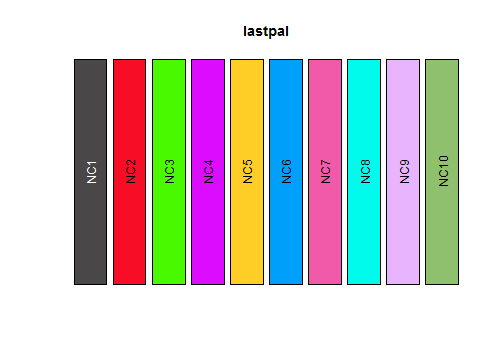Although the Polychrome package ships with several pre-built multicolored palettes, it also contains tools to build more. In this vignette, we expain how to use the createPalette function for this purpose.

# Getting Started

``````library(Polychrome)
``````

Here are the arguments of the main function.

``````args(createPalette)
``````
``````## function (N, seedcolors, prefix = "NC", range = c(30, 90), target = c("normal",
##     "protanope", "deuteranope", "tritanope"), M = 50000)
## NULL
``````
• N is the number of colors to include in the palette
• seedcolors is a list of one or more starting colors that you would like included in the palette.
• prefix is a character string used to create default names for the palette colors.
• range is a numeric vector of length 2 indicating the minimum and maximum desired luminance for the palette.
• M is an an integer representing the number of random colors to be generated in order to create the palette.

## Recommendations

1. The seed colors usually have a small effect on the overall palette, but they can change the order. White and black appear to be exceptions; they tend not to be included in the palette unless forced. One reason to use more seed colors would be to aumgent an existing small palette by adding distinguishable colors.
2. We show below that an upper bound on a good set of colors is likely to be around 40. It may be better in the long run to build a palette that you like of about this size, and then just use colors from the beginning if you don't need that many.
3. The speed of the algorithm mainly depends on M. You get a richer selection of colors with larger M. We have been successful using the default of 50000.
4. The range depends on whether you expect to use a light background (take range = c(10, 60)), a dark background (take range = c(55, 95) )or both (take range = c(30, 80)).
5. Unless you really need colors called X1, …, X37, you are probably better off using the colorNames function to assign meaningful names after creating the palette.

# A Small Palette

``````set.seed(723451) # for reproducibility
firstpal <- createPalette(10, c("#010101", "#ff0000"), M=1000)
firstpal
``````
``````##       NC1       NC2       NC3       NC4       NC5       NC6       NC7       NC8
## "#224F63" "#FF0D45" "#F0E716" "#FD2EE3" "#2E51FB" "#38F7B2" "#FC9BA4" "#D39CF8"
##       NC9      NC10
## "#0DEAFB" "#D7DDBE"
``````

The probably surprising result is that the seed colors are actually not included in the final palette. There are two reasons for this. First, the colors 'black' and 'red' are outside the specified (default) luminance range. So, they are replaced by the closest randomly generated colors that are inside the range. But that leads us to the second reason. We specified a small value of M. The algorithm operates by randomly selecting a set of potential colors in the desired range, and will eventually assemble the palette from this set. With only 1000 candidates, there may not be anything that looks close to pure red or pure black in the set of candidates.

``````swatch(firstpal)
``````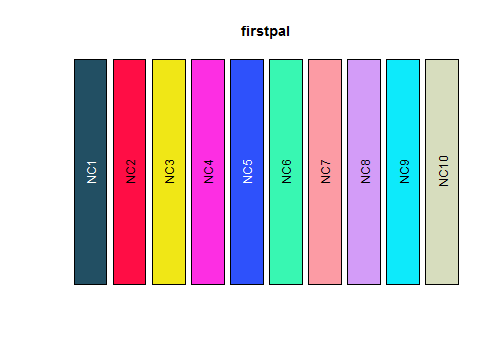## Do the seeds matter?

Here is the palette that we get if we just start with the color black. Note that we keep resetting the random seed – the one for the random number generator – to make sure we are choosing from the same set of randomly generated candidates.

``````set.seed(723451) # for reproducibility
second <- createPalette(10, c("#010101"), M=1000)
swatch(second)
``````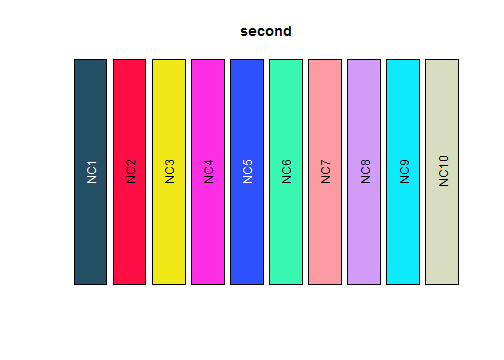This palette is essentially indistinguishable from the first one. However, here is what happens if we just start with the color red.

``````set.seed(723451) # for reproducibility
third <- createPalette(10, c("#ff0000"), M=1000)
swatch(third)
``````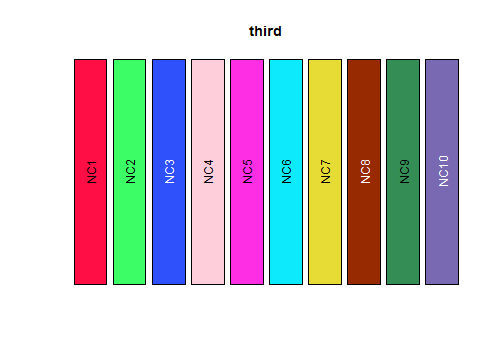The red, green, and blue triumvirate stays near the top of the list, but black has disappeared. What happens if we instead start with green and blue?

``````set.seed(723451) # for reproducibility
fourth <- createPalette(10, c("#00ff00", "#0000ff"), M=1000)
swatch(fourth)
``````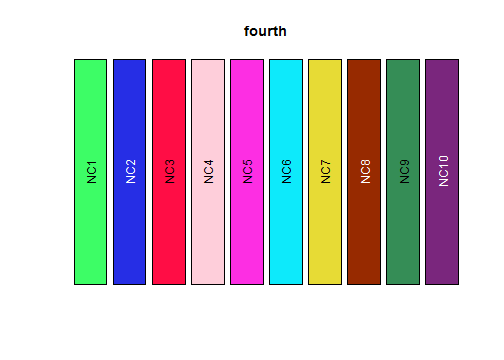Except for the forced reordering at the start, we get nearly the same palette. So, let's instead try starting with cyan, magenta, and yellow.

``````set.seed(723451) # for reproducibility
fifth <- createPalette(10, c("#00ffff", "#ff00ff", "#ffff00"), M=1000)
swatch(fifth)
``````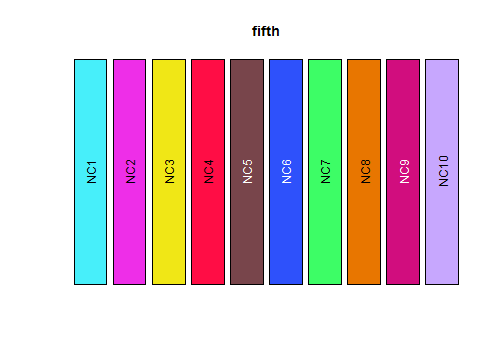We see that the initial seed colors have a relatively small effect. This result should not be unexpected based on the “greedy” nature of the underlying algorithm, which tries at each step to find the color that is most distant from everything already included in the list. The vertices of the polyhedron in three-dimensional LUV space include the usual primary colors.

# How many good colors can we find?

Now we'd like to examine how big we think we can realistically make N, the number of distinguishable colors. We can estimate this by first estimating the volume of the visible region of LUV space. We can start by determining the convex hull of the UV projection. We saw in the manuscript that our 36-color palette uses almost all of the visible region. So (for speed as well as simplicity), we are going to work with that palette.

First, we have to get the LUV coordinates of the colors.

``````library(colorspace)
p36 <- palette36.colors(36)
luvmat <- as(hex2RGB(p36), "LUV")
y <- luvmat@coords
``````

Now we can use a function from the grDevices package to compute the convex hull.

``````hull <- y[chull(y[,2], y[,3]), 2:3]
uvscatter(p36)
polygon(hull[,1], hull[,2], lwd=2)
``````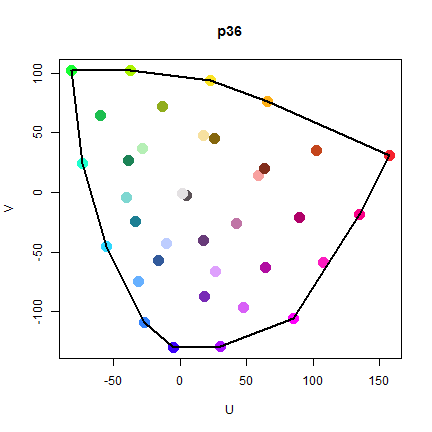The next step is to compute the area of the convex hull. While there are R packages that include functions to do this computation, the algorithm is sufficiently easy that it hardly seems worth it to load another package.

``````hullaug <- rbind(hull, hull[1,])
N <- nrow(hullaug)
fst <-sum(hullaug[1:(N-1), 1]*hullaug[2:N, 2] )
snd <- sum(hullaug[2:N, 1]*hullaug[1:(N-1), 2] )
area <- (snd - fst)/2
area
``````
``````##  37424.93
``````

So, a crude (over)estimate of the volume of the viewable region of LUV space is to take the range of L values (0–100) and multiple it times the UV area. This is an overestimate for two reasons. First, we can't actually use the entire viewable range of luminances agains any single background, so the factor of 100 is probably closer to 60. Second, not every combination of L-U-V values in this region designates an actual color.

``````overvol <- 100*area
overvol
``````
``````##  3742493
``````

Now, we want colors to be 40 units apart to be reliably distinguishable by most viewers. So, each time we pick a color, it should “occupy” a sphere of radius 20 to avoid picking indistinguishable colors. The volume of such a sphere is:

``````sphere <- 4/3*pi*20^3
sphere
``````
``````##  33510.32
``````

To get the most generous (over)estimate of the number of colors, we pretend for a moment that we can pack spheres into the space with minimal waste. By the Kepler conjecture, we know that this means that the spheres can occupy about 74% of the volume.

``````100 * area/sphere *0.74
``````
``````##  82.64453
``````
``````60 * area/sphere *0.74
``````
``````##  49.58672
``````

If we could use the full range of luminances, we could get about 82 colors. If we can only use a range of luminance values about 60 units wide, then we can at most hope to get 50 distinct colors.

### Improving the estimate

We can empirically improve our estimate of the volume of the visible portion of LUV space. We start by generating a tight, regularly spaced grid of values.

``````L <- 0:100
U <- -60:150
V <- -150:150
luv <- cbind(L = rep(L, length(U)*length(V)),
U = rep(rep(U, each=length(L)), times=length(V)),
V = rep(V, each=length(L)*length(U)))*1.0
``````

Next, we convert the LUV coordinates into hexadecimal representations in RGB space.

``````tested <- LUV(luv)
co <- tested@coords
``````

Whenever the conversion fails (yielding an NA value), the LUV coordinates were not visible. So, we can estimate the fraction of the regular grid that is visible:

``````he <- hex(tested)
summary(is.na(he))
``````
``````##    Mode   FALSE    TRUE
## logical 1381103 5033508
``````
``````mean(!is.na(he))
``````
``````##  0.2153058
``````

That is roughly 20%. Now we look at how many spheres we could pack into this space.

``````VOL <- diff(range(L)) * diff(range(U)) * diff(range(V)) * 0.205
VOL
``````
``````##  1291500
``````
``````VOL/sphere * 0.74
``````
``````##  28.51987
``````

Hmm. This computation suggest that we can only find about 28 distinguishable colors. Since we have examples of palettes with more colors than this, we must now somehow be underestimating the value.

But it's clear why. Many of the spheres can be placed on the boundary, that is, along the faces, edges, or even vertices of the polyhedron. In that case, half or more of their volume sticks outsde the polyhedron. If we crudely estimate that half of the volume of half of the spheres can be ignored, then this adjustment roughly undoes the correction for the sphere packing density.

``````VOL/sphere
``````
``````##  38.54036
``````

So, our final estimate is that the maximum number of distinguishable colors is roughly 38 or 39. Being generous (and tending to favor round numbers), we're going to take it to be about 40.

# Computational Complexity

The final parameter we want to look at is M, the number of candidate colors that we search through. In general, we expect the time needed to run the algorithm to be approximately N*M, since we must search through all M candidates for each of the N desired colors. The trade-off, of course, is that we can find more colors that are distinguishable with more candidates. Here we increase M from 1000 to 10000.

``````set.seed(723451) # for reproducibility
lastpal <- createPalette(10, c("#010101", "#ff0000"), M=10000)
lastpal
``````
``````##       NC1       NC2       NC3       NC4       NC5       NC6       NC7       NC8
## "#494747" "#F70D26" "#49F900" "#DC0DFF" "#FFCE26" "#009FF9" "#F05AA8" "#00FBEC"
##       NC9      NC10
## "#E8B4FE" "#8EC06E"
``````
``````swatch(firstpal)
````````````swatch(lastpal)
``````